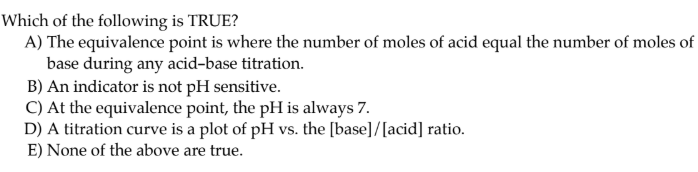# Which of the following is TRUE? A) The equivalence point is where the number of moles of acid equal the number of moles of base during any acid-base titration. B) An indicator is not pH sensitive. C) At the equivalence point, the pH is always 7. D) A titration curve is a plot of pH vs. the [base]/[acid] ratio. E) None of the above are true.